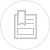# analysis of the adsorbent capacity of a carbon

## granulometry of an powdered activated carbon ( PAC )

Dry a sample of powdered activated carbon for 4 hours at 120 °C. Precisely weigh 10g and place on a first screen (125 µm). Pass the screen under a stream of water flowing at a moderate pressure to wash the carbon that remains on the screen. This task is conducted over a white enamelled container and the wash is pursued until no more carbon passes through the screen. Then place the screen in an oven for 4 hours at 120°C. Weigh the screen remains. Use the difference in relation to the initial figure recorded to calculate the amount of activated carbon that has passed through the screen. Express this ratio as a percentage.

Repeat these steps with smaller mesh screens (90-63-45 µm).

## adsorption isotherm = Freudlich isotherm

The adsorption capacity of an activated carbon can be estimated for a given pollutant using an adsorption isotherm.

The Freundlich model (see adsorption) establishes the relationship between the weight of pollutant absorbed per unit weight of carbon

and the aqueous concentration Ce of pollutant at equilibrium with the activated carbon.

K and n, two coefficients established through experimentation.

These isotherm curves (see figure 25) can be used to establish the following properties for an activated carbon:

• the maximum adsorption capacity that can be used to estimate the maximum weight of pollutant adsorbed at equilibrium per unit weight of carbon. This maximum adsorption capacity is valid for an aqueous pollutant concentration equal to the initial concentration of the pollution;
• the adsorption index (Q10) for a specific pollutant is defined as the weight of pollution adsorbed per unit weight of activated carbon for an aqueous concentration of pollutant equal to one tenth of the pollutant initial concentration. This index is used to estimate the adsorption capacity of activated carbon under average conditions that are closer to the normal operation of the carbon in a fixed bed system.

If these capacities and indices are used as basis to compare different suppliers and/or reception methods for activated carbon an agreement should be reached first with the various suppliers on a very precise method of operation.

### plotting an isotherm

• take six 1.2 liters glass flasks;
• add 1 liter of water containing the pollutant that is under investigation. (note: when dealing with a volatile pollutant, the flasks should be filled to the brim to avoid losing any amount by volatility);
• when studying a naturally polluted water, add the water as provided;
• when studying a simulated water, as a rule 1mg of the pollutant tested should be injected into each flask. This applies to studies designed to assess the potability of water (note: the same applies to industrial WW but adding 10 – 100 – 1,000 mg per flask depending on the waste being simulated);
• reduce the carbon under examination to a powder by crushing it in a mortar and dry-sieving it through a 40 µm screen. Collect the particles that have passed through the screen;
• dry the carbon for 4 hours at 120°C in a powder box;
• add increasingly higher amounts of activated carbon into the flasks containing the polluted water. Ensure that the amounts are precisely weighted.

After a minimum of 5 days at moderate agitation (30 to 40 rpm) and constant temperature, filter each sample through a 0.45 µm cellulose acetate membrane. Discard the first 100 millilitres of filtrate and measure the pollutant present in the remaining filtrate. Therefore, for each amount of activated carbon the aqueous concentration of pollutant at equilibrium is obtained.

Plot the isotherm curve using log-log coordinates. Compute the concentration at equilibrium expressed in mg · L–1 on the X-axis and the weight of pollutant adsorbed per unit weight of carbon on the Y-axis (figure 26).

### kinetics study

In the case of granular activated carbon, proper contact time can also be achieved without the need to crush the activated carbon. This is achieved via continuous agitation for an extended period of time (several weeks). Measuring the pollutant concentration at equilibrium several times during the contact period can be used to model the adsorption of the pollutant concerned.

## carbon ash

Precisely weigh 1 g of dry carbon and place in a crucible; P1 is weight.

Incinerate the activated carbon tested at 625 °C (± 25 °C). Thoroughly check for complete incineration. After cooling, weigh the ashes. If P2 is the weight obtained, the ash content C is expressed as follows.

## iodine index

Based on the iodine isotherm for the activated carbon concerned the iodine index represents the iodine weight adsorbed per unit weight of carbon for a 0.02 N residual iodine concentration in the filtrate.

## dechlorination capacity

The dechlorination capacity of an activated carbon is defined as the depth of the bed required to reduce the amount of existing chlorine by half at a percolation rate of 20 m · h–1.

Boil the activated carbon in distilled water to expel any air that it may contain. Place the wet carbon away from air in a 22 mm diameter tube until a precise 10 cm high column is obtained.

Using a 7.5 pH sodium hypochlorite solution, prepare a chlorinated water solution containing 10 mg · L–1 of active chlorine.

Percolate this chlorinated water through the carbon column at a rate of 20 m · h–1. After 30 minutes operation, precisely titrate the chlorinated water at the column intake (a expressed in mg · L–1) and at the column outlet (b mg · L–1).

H being the layer depth in cm, calculate the half-dechlorination length G (in cm):